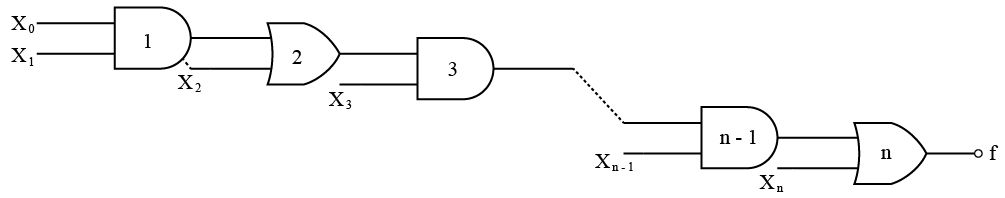# ISRO Scientist or Engineer Computer Science 2008

Instructions

For the following questions answer them individually

Question 11

# The Boolean theorem $$AB + \overline{A} C + BC = AB + \overline{A} C$$ crresponds toQuestion 12

# In the given network of AND and OR gates f can be written asQuestion 13

# If $$N^{2}= (7601)_{g}$$ , where N is a positive integer, then the value of NQuestion 14

# Assumethat each character code consists of 8 bits. The number of characters that can be transmitted per second through an synchronousserialline at 2400 baudrate, andwith two stop bits isQuestion 15

# Four jobs to be executed on a single processor system arrive at time O in the order A, B, C, D. There burst CPU time requirements are 4,1, 8, 1, time units respectively. Thecompletion time of A under robin round scheduling with time slice of one time unit isQuestion 16

# Which one of the following algorithm design techniques is used in finding all pairs of shortest distances in a graph?Question 17

# The address space of 8086 CPU isQuestion 18

# More than one wordare put in one cache block toQuestion 19

# The performanceofa pipelined processorsuffers ifQuestion 20

# If $$(12 x)_{3} = (123)_{x}$$, then the value of x isOR